### Problem Set 2 Pythagoras Theorem Class 10th Mathematics Part 2 MHB Solution

Problem Set 2

1. Out of the following which is the Pythagorean triplet? Some questions and their…
2. In a right-angled triangle, if sum of the squares of the sides making right angle is…
3. Out of the dates given below which date constitutes a Pythagorean triplet? Some…
4. If a, b, c are sides of a triangle and a2 + b2 = c2, name the type of triangle. Some…
5. Find perimeter of a square if its diagonal is 10 2 cm. Some questions and their…
6. Altitude on the hypotenuse of a right angled triangle divides it in two parts of…
7. Height and base of a right angled triangle are 24 cm and 18 cm find the length of its…
8. In D ABC, AB = 6 root {3} cm, AC = 12 cm, BC = 6 cm. Find measure of angle a . Some…
9. Find the height of an equilateral triangle having side 2a. Solve the following…
10. Do sides 7 cm , 24 cm, 25 cm form a right angled triangle ? Give reason. Solve the…
11. Find the length a diagonal of a rectangle having sides 11 cm and 60cm. Solve the…
12. Find the length of the hypotenuse of a right angled triangle if remaining sides are 9…
13. A side of an isosceles right angled triangle is x. Find its hypotenuse. Solve the…
14. In ∆ PQR; PQ = root {8} 8 , QR = root {5} , PR = root {3} Is ∆ PQR a right angled…
15. In deltarat , angle s = 90^{degree } , anglet = 30^{circ} , rt = 12cm then find RS…
16. Find the diagonal of a rectangle whose length is 16 cm and area is 192 sq.cm.…
17. Find the length of the side and perimeter of an equilateral triangle whose height is…
18. In triangle abc seg AP is a median. If BC = 18, AB2 + AC2 = 260 Find AP.…
19. ∆ ABC is an equilateral triangle. Point P is on base BC such that pc = {1}/{3} bc…
20. From the information given in the figure 2.31, prove that PM = PN = root {3} x a…
21. Prove that the sum of the squares of the diagonals of a parallelogram is equal to the…
22. Pranali and Prasad started walking to the East and to the North respectively, from the…
23. In deltaabc , angle bac = 90^{degree } seg BL and seg CM are medians of ∆ ABC.…
24. Sum of the squares of adjacent sides of a parallelogram is 130 sq.cm and length of one…
25. In ∆ ABC, seg AD seg BC DB = 3CD. Prove that : 2AB2 = 2AC2 + BC2…
26. In an isosceles triangle, length of the congruent sides is 13 cm and its base is 10…
27. In a trapezium ABCD, seg AB || seg DC seg BD seg AD, seg AC seg BC, If AD = 15, BC =…
28. In the figure 2.35, ∆ P20QR is an equilatral triangle. Point S is on seg QR such that…
29. Seg PM is a median of ∆ PQR. If PQ = 40, PR = 42 and PM = 29, find QR.…
30. Seg AM is a median of ∆ ABC. If AB = 22, AC = 34, BC = 24, find AM…

###### Problem Set 2
Question 1.

Some questions and their alternative answers are given. Select the correct alternative.

Out of the following which is the Pythagorean triplet?

A. (1, 5, 10)

B. (3, 4, 5)

C. (2, 2, 2)

D. (5, 5, 2)

A Pythagorean triplet consists of three positive integers (l, b, h) such that

l2 + b2 = h2

and (3, 4, 5) is a Pythagorean triplet as,

52 = 32 + 42

Question 2.

Some questions and their alternative answers are given. Select the correct alternative.

In a right-angled triangle, if sum of the squares of the sides making right angle is 169 then what is the length of the hypotenuse?

A. 15

B. 13

C. 5

D. 12

Given,

Sum of the squares of the sides making right angle = 169

⇒ (base)2 + (perpendicular)2 = 169

But we know, By Pythagoras's theorem

(Hypotenuse)2 = (base)2 + (Perpendicular)2

⇒ (Hypotenuse)2 = 169

⇒ Hypotenuse = 13 units.

Question 3.

Some questions and their alternative answers are given. Select the correct alternative.

Out of the dates given below which date constitutes a Pythagorean triplet?

A. 15/08/17

B. 16/08/16

C. 3/5/17

D. 4/9/15

A Pythagorean triplet consists of three positive integers (l, b, h) such that

l2 + b2 = h2

and 15/08/17 is a Pythagorean triplet as,

152 + 82 = 172

i.e. 225 + 64 = 289

Question 4.

Some questions and their alternative answers are given. Select the correct alternative.

If a, b, c are sides of a triangle and a2 + b2 = c2, name the type of triangle.

A. Obtuse angled triangle

B. Acute angled triangle

C. Right angled triangle

D. Equilateral triangle

As, the sides of right-angled triangles satisfies the Pythagoras theorem, i.e.

(Hypotenuse)2 = (base)2 + (Perpendicular)2

We know that,

Diagonal of a square = √2 a

Where 'a' is the side of the triangle.

⇒ √2 a = 10√2

⇒ a = 10 cm

Also, we know

Perimeter of square = 4a

Where 'a' is the side of the triangle

∴ Perimeter of given square = 4(10) = 40 cm

Question 6.

Some questions and their alternative answers are given. Select the correct alternative.

Altitude on the hypotenuse of a right angled triangle divides it in two parts of lengths 4 cm and 9 cm. Find the length of the altitude.

A. 9 cm

B. 4 cm

C. 6 cm

D.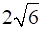cm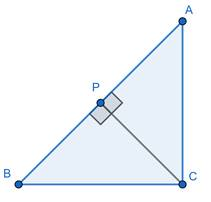Let ABC be a right-angled triangle, at B, and BP be the altitude on hypotenuse that divides it in two parts such that,

AP = 4 cm

PC = 9 cm

As, ABC, ABP and CBP are right-angled triangles, therefore they all satisfy Pythagoras theorem i.e.

(Hypotenuse)2 = (base)2 + (Perpendicular)2

∴ In ΔABC

AB2 + BC2 = AC2

⇒ AB2 + BC2 = (AP + CP)2

⇒ AB2 + BC2 = (4 + 9)2 = 132

⇒ AB2 + BC2 = 169 

∴ In ΔABP

AP2 + BP2 = AB2

AP2 + 42 = AB2 

∴ In ΔCBP

CP2 + BP2 = BC2

⇒ 92 + BP2 = BC2 

Adding  and , we get

AP2 + 42 + 92 + BP2 = AB2 + BC2

⇒ 2AP2 + 16 + 81 = 169 [From 1]

⇒ 2AP2 = 72

⇒ AP2 = 36

⇒ AP = 6 cm

Hence, length of Altitude is 6 cm.

Question 7.

Some questions and their alternative answers are given. Select the correct alternative.

Height and base of a right angled triangle are 24 cm and 18 cm find the length of its hypotenuse

A. 24 cm

B. 30 cm

C. 15 cm

D. 18 cm

By Pythagoras theorem

(Hypotenuse)2 = (base)2 + (Perpendicular)2

Given,

Base = 18 cm

Perpendicular = Height = 24 cm

⇒ Hypotenuse2 = 242 + 182

⇒ Hypotenuse2 = 576 + 324

⇒ Hypotenuse2 = 900

⇒ Hypotenuse = 30 cm

Question 8.

Some questions and their alternative answers are given. Select the correct alternative.

In D ABC, AB =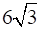cm, AC = 12 cm, BC = 6 cm. Find measure of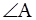.

A. 30°

B. 60°

C. 90°

D. 45°

As,

(6√3)2 + 62 = 122

⇒ AB2 + BC2 = AC2

i.e. sides of the triangle ABC satisfy the Pythagoras theorem

(Hypotenuse)2 = (base)2 + (Perpendicular)2

∴ ABC is a right-angled triangle with hypotenuse as AC

Now,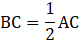By converse of 30°-60°-90° triangle theorem i.e.

In a right-angled triangle, if one side is half of the hypotenuse then the angle

opposite to that side is 30°.

∠A = 30°

Question 9.

Solve the following examples.

Find the height of an equilateral triangle having side 2a.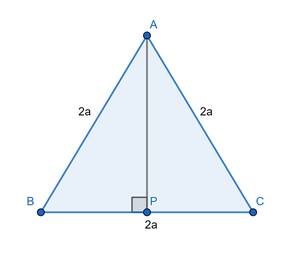Let ABC be an equilateral triangle,

Let AP be a perpendicular on side BC from A.

To find : Height of triangle = AP

As, ABC is an equilateral triangle we have

AB = BC = CA = 2a

Also, we know that Perpendicular from a vertex to corresponding side in an equilateral triangle bisects the side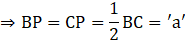Now, In ΔABP, By Pythagoras theorem

(Hypotenuse)2 = (base)2 + (Perpendicular)2

⇒ AB2 = BP2 + AP2

⇒ (2a)2 = a2 + AP2

⇒ AP2 = 4a2 - a2

⇒ AP2 = 3a2

⇒ AP = a√3

Question 10.

Solve the following examples.

Do sides 7 cm , 24 cm, 25 cm form a right angled triangle ? Give reason.

Yes,

Because

72 + 242 = 252 [i.e. 49 + 576 = 625]

As, sides satisfy the Pythagoras theorem, i.e.

(Hypotenuse)2 = (base)2 + (Perpendicular)2

They do form a right-angled triangle.

Question 11.

Solve the following examples.

Find the length a diagonal of a rectangle having sides 11 cm and 60cm.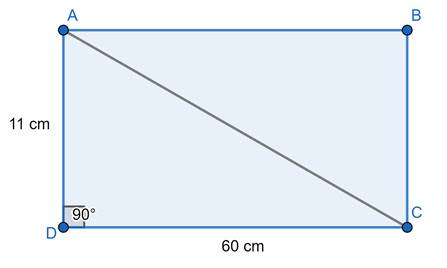Let ABCD be a rectangle, with

AB = CD = 60 cm

BC = DA = 11 cm

And AC be a diagonal.

As, ∠A = 90°

ADC is a right-angled triangle, By Pythagoras Theorem i.e.

(Hypotenuse)2 = (base)2 + (Perpendicular)2

AC2 = (CD)2 + (DA)2

⇒ AC2 = 602 + 112

⇒ AC2 = 3600 + 121

⇒ AC2 = 3721

⇒ AC = 61 cm

Question 12.

Solve the following examples.

Find the length of the hypotenuse of a right angled triangle if remaining sides are 9 cm and 12 cm.

In a right-angled triangle

By Pythagoras theorem

(Hypotenuse)2 = (base)2 + (Perpendicular)2

Given,

Other sides are 9 cm and 12 cm

⇒ Hypotenuse2 = 92 + 122

⇒ Hypotenuse2 = 81 + 144

⇒ Hypotenuse2 = 225

⇒ Hypotenuse = 15 cm

Question 13.

Solve the following examples.

A side of an isosceles right angled triangle is x. Find its hypotenuse.

In a right-angled triangle

By Pythagoras theorem

(Hypotenuse)2 = (base)2 + (Perpendicular)2

As, the triangle is isosceles

Base = Perpendicular = x

[Hypotenuse can't be equal to any of the sides, because hypotenuse is the greatest side in a right-angled triangle and it must be greater than other two sides]

⇒ (Hypotenuse)2 = x2 + x2

⇒ (Hypotenuse)2 = 2x2

⇒ Hypotenuse = x√2

Question 14.

Solve the following examples.

In ∆ PQR; PQ =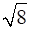8 , QR =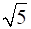, PR =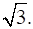Is ∆ PQR a right angled triangle? If yes, which angle is of 90°?

As,

(√5)2 + (√3)2 = (√8)2

⇒ QR2 + PR2 = PQ2

i.e. sides of the triangle ABC satisfy the Pythagoras theorem

(Hypotenuse)2 = (base)2 + (Perpendicular)2

∴ PQR is a right-angled triangle at R [As hypotenuse is PQ].

Question 15.

In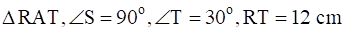then find RS and ST.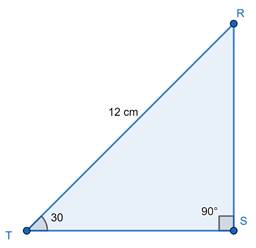As, ∠S = 90°, and ∠T = 30° and RT = 12 cm is given.

Clearly, RTS is a 30°-60°-90° triangle.

We know, Property of 30°-60°-90° triangle i.e.

If acute angles of a right angled-triangle are 30° and 60°, then the side opposite

30° angle is half of the hypotenuse and the side opposite to 60° angle is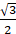times of hypotenuse.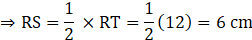And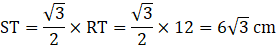Question 16.

Find the diagonal of a rectangle whose length is 16 cm and area is 192 sq.cm.

Given,

Length of rectangle, l = 16 cm

Area of rectangle = length × breadth

⇒ 192 = 16b

⇒ b = 12 cm

Also, we know that

Length of diagonal = √(l2 + b2)

Where, l = length and b = breadth

⇒ length of diagonal = √(162 + 122)

= √(256 + 144) = 20 cm

Question 17.

Find the length of the side and perimeter of an equilateral triangle whose height is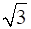cm.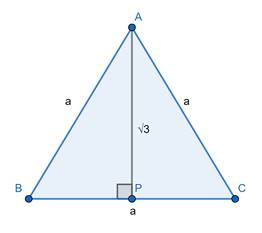Let ABC be an equilateral triangle,

Let AP be a perpendicular on side BC from A.

To find : Height of triangle = AP

As, ABC is an equilateral triangle we have

AB = BC = CA = 'a'

Also, we know that Perpendicular from a vertex to corresponding side in an equilateral triangle bisects the side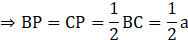Now, In ΔABP, By Pythagoras theorem

(Hypotenuse)2 = (base)2 + (Perpendicular)2

⇒ AB2 = BP2 + AP2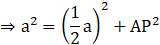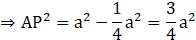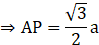Given,

Height = √3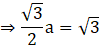⇒ a = 2 cm

Also, Perimeter of equilateral triangle = 3a

Where 'a' depicts side of equilateral triangle.

∴ Perimeter = 3(2) = 6 cm

Question 18.

In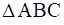seg AP is a median. If BC = 18, AB2 + AC2 = 260 Find AP.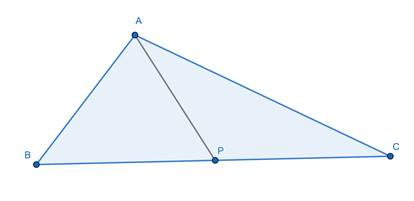We know, By Apollonius theorem

In ΔABC,

if P is the midpoint of side BC, then AB2 + AC2 = 2AP2 + 2BP 2

Given that, AP is median i.e. P is the mid-point of BC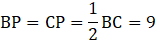And BC = 18 cm

and AB2 + AC2 = 260

⇒ 260 = 2AP2 + 2(9)2

⇒ 260 = 2AP2 + 162

⇒ 98 = 2AP2

⇒ AP2 = 49

⇒ AP = 7 units

Question 19.

∆ ABC is an equilateral triangle. Point P is on base BC such that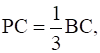if AB = 6 cm find AP.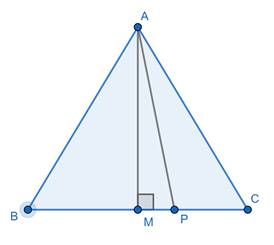ABC be an equilateral triangle,

Point P is on base BC, such that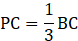Let us construct AM perpendicular on side BC from A.

As, ABC is an equilateral triangle we have

AB = BC = CA = 6 cm

Also, we know that Perpendicular from a vertex to corresponding side in an equilateral triangle bisects the side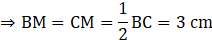Now, In ΔACM, By Pythagoras theorem

(Hypotenuse)2 = (base)2 + (Perpendicular)2

⇒ CA2 = CM2 + AM2

⇒ (6)2 = (3)2 + AM2

⇒ 36 = 9 + AM2

⇒ AM2 = 27 

As,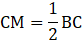We have,

CM - PC = PM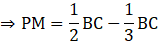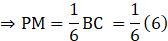⇒ PM = 1 cm

Now, In right angled triangle AMP, By Pythagoras theorem

(AP)2 = (AM)2 + (PM)2

⇒ (AP)2 = 27 + 12

⇒ AP2 = 28

⇒ AP = 2√7 cm

Question 20.

From the information given in the figure 2.31, prove that PM = PN =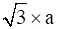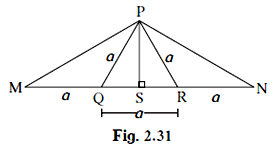In ΔPQS and ΔPSR, By Pythagoras theorem

i.e. (Hypotenuse)2 = (base)2 + (Perpendicular)2

PQ2 = QS2 + PS2 

PR2 = SR2 + PS2 

Subtracting  from ,

PQ2 - PR2 = QS2 - SR2

⇒ a2 - a2 = QS2 - SR2

⇒ QS2 = SR2

⇒ QS = SR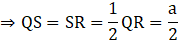Also,

MS = MQ + QS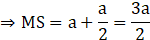And

SN = SR + RN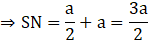In ΔPSM and ΔPSN, By Pythagoras theorem

PM2= PS2 + MS2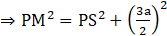PN2= PS2 + SN2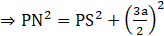From  and 

PM2 = PN2

⇒ PM = PN

Hence Proved.

Question 21.

Prove that the sum of the squares of the diagonals of a parallelogram is equal to the sum of the squares of its sides.

Let ABCD be a parallelogram, with AB = CD ; AB || CD and BC = AD ; BC || AD.

Construct AE ⊥ CD and extend CD to F such that, BF ⊥ CF.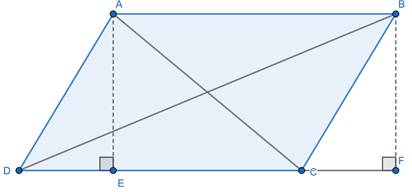In ΔAED and ΔBCF

AE = BF [Distance between two parallel lines i.e. AB and CD]

AD = BC [opposite sides of a parallelogram are equal]

∠AED = ∠BFC [Both 90°]

ΔAED ≅ ΔBCF [By Right Angle - Hypotenuse - Side Criteria]

⇒ DE = CF [Corresponding sides of congruent triangles are equal] 

In ΔBFD, By Pythagoras theorem i.e.

(Hypotenuse)2 = (base)2 + (Perpendicular)2

BD2 = DF2 + BF2

⇒ BD2 = (CD + CF)2 + BF2 

In ΔAEC, By Pythagoras theorem

AC2 = AE2 + CE2

⇒ AC2 = AE2 + (CD - AE)2

⇒ AC2 = BF2 + (CD - CF)2 [As, AE = BF and CF = AE] 

In ΔBCF, By Pythagoras theorem,

BC2 = BF2 + CF2

BF2 = BC2 - CF2 

BD2 + AC2 = 2BF2 + (CD + CF)2 + (CD - CF)2

⇒ BD2 + AC2= 2BC2 - 2CF2 + CD2 + CF2 + 2CD.CF + CD2 + CF2 - 2CD.CF

⇒ BD2 + AC2 = 2BC2 + 2CD2

⇒ BD2 + AC2 = BC2 + BC2 + CD2 + CD2

⇒ BD2 + AC2 = AB2 + BC2 + CD2 + AD2 [since BC = AD and AB = CD]

Hence, the sum of the squares of the diagonals of a parallelogram is equal to the sum of the squares of its sides.

Question 22.

Pranali and Prasad started walking to the East and to the North respectively, from the same point and at the same speed. After 2 hours distance between them was 15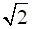km. Find their speed per hour.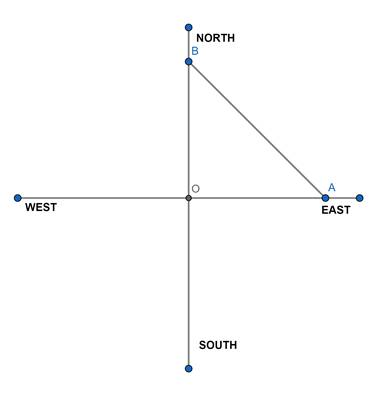Let their speed be 'x' km/h

We know, distance = speed × time

In two hours,

Distance travelled by both = '2x' km

Let their starting point be 'O', and Pranali and Prasad reach the point A in the East and point B in the north direction respectively.

Clearly, AOB is a right-angled triangle, So By Pythagoras theorem

(Hypotenuse)2 = (base)2 + (Perpendicular)2

(AB)2 = (OA)2 + (OB)2

As, AB = distance between them = 15√2 km

And OA = OB = distance travelled by each = 2x

⇒ (15√2)2 = (2x)2 + (2x)2

⇒ 450 = 8x2

⇒ x2 = 56.25

⇒ x = 7.5 km/h

Question 23.

In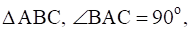seg BL and seg CM are medians of ∆ ABC. Then prove that : 4(BL2 + CM2) = 5 BC2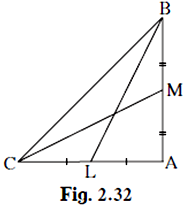We know, By Apollonius theorem

In ΔABC, if L is the midpoint of side AC, then AB2 + BC2 = 2BL2 + 2AL 2

Given that, BL is median i.e. L is the mid-point of CA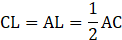⇒ AB2 + BC2 = 2BL2 + 2AL2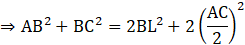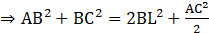Also, if M is the midpoint of side AB, then AC2 + BC2 =2CM2 + 2BM2

Given that, CM is median i.e. M is the mid-point of BA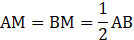⇒ AC2 + BC2 = 2CM2 + 2BM2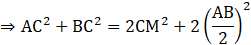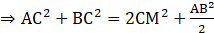Also, In ΔABC, By Pythagoras theorem i.e.

(Hypotenuse)2 = (base)2 + (Perpendicular)2

⇒ BC2 = AC2 + AB2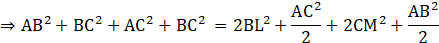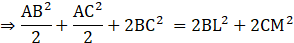⇒ AB2 + AC2 + 4BC2 = 4(BL2 + CM2)

⇒ BC2 + 4BC2 = 4(BL2+ CM2) [From 3]

⇒ 5BC2 = 4(BL2+ CM2)

Hence Proved.

Question 24.

Sum of the squares of adjacent sides of a parallelogram is 130 sq.cm and length of one of its diagonals is14 cm. Find the length of the other diagonal.

Let ABCD be a parallelogram, with AB = CD ; AB || CD and BC = AD ; BC || AD.

Construct AE ⊥ CD and extend CD to F such that, BF ⊥ CF.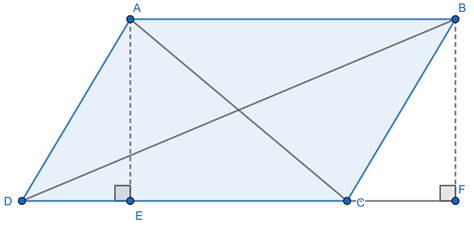Given: sum of squares of adjacent side = 130

⇒ CD2 + BC2 = 130 and

Length of one diagonal = 14 cm [let it be AC]

To Find: length of the other diagonal, BD

In ΔAED and ΔBCF

AE = BF [Distance between two parallel lines i.e. AB and CD]

AD = BC [opposite sides of a parallelogram are equal]

∠AED = ∠BFC [Both 90°]

ΔAED ≅ ΔBCF [By Right Angle - Hypotenuse - Side Criteria]

⇒ DE = CF [Corresponding sides of congruent triangles are equal] 

In ΔBFD, By Pythagoras theorem i.e.

(Hypotenuse)2 = (base)2 + (Perpendicular)2

BD2 = DF2 + BF2

⇒ BD2 = (CD + CF)2 + BF2 

In ΔAEC, By Pythagoras theorem

AC2 = AE2 + CE2

⇒ AC2 = AE2 + (CD - AE)2

⇒ AC2 = BF2 + (CD - CF)2 [As, AE = BF and CF = AE] 

In ΔBCF, By Pythagoras theorem,

BC2 = BF2 + CF2

BF2 = BC2 - CF2 

BD2 + AC2 = 2BF2 + (CD + CF)2 + (CD - CF)2

⇒ BD2 + AC2= 2BC2 - 2CF2 + CD2 + CF2 + 2CD.CF + CD2 + CF2 - 2CD.CF

⇒ BD2 + AC2 = 2BC2 + 2CD2

⇒ BD2 + 142 = 2(130)

⇒ BD2 + 196 = 260 [Using given data]

⇒ BD2 = 64

⇒ BD = 8 cm

Hence, length of other diagonal is 8 cm.

Question 25.

In ∆ ABC, seg AD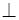seg BC DB = 3CD. Prove that : 2AB2 = 2AC2 + BC2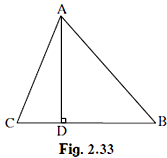Given,

DB = 3CD

Also,

BC = CD + DB = CD + 3CD

⇒ BC = 4CD 

As, AD ⊥ BC, By Pythagoras theorem i.e.

(Hypotenuse)2 = (base)2 + (Perpendicular)2

In Δ ACD

AC2 = AD2 + CD2 

In ΔABD

⇒ AB2 = AD2 + (3CD)2

⇒ AB2 = AD2 + 9CD2 

Subtracting  from 

⇒ AB2 - AC2 = 9CD2 - CD2

⇒ AB2 = AC2 + 8CD2

⇒ 2AB2 = 2AC2 + 16CD2

⇒ 2AB2 = 2AC2 + (4CD)2

⇒ 2AB2 = 2AC2 + BC2 [From 1]

Hence Proved.

Question 26.

In an isosceles triangle, length of the congruent sides is 13 cm and its base is 10 cm. Find the distance between the vertex opposite the base and the centroid.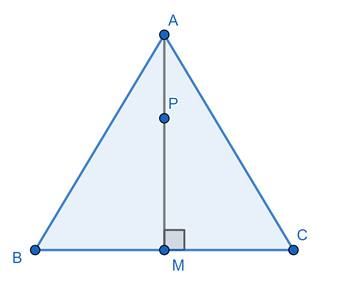Let ABC be an isosceles triangle, In which AB = AC = 13 cm

And BC = 10 cm

Let AM be median on BC such that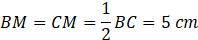Let P be centroid on median BC

To Find : AP [Distance between vertex opposite the base and centroid]

We know, By Apollonius theorem

In ΔABC, if M is the midpoint of side BC, then AB2 + AC2 = 2AM2 + 2BM 2

Putting values, we get

(13)2 + (13)2 = 2AM2 + 2(5)2

⇒ 169 + 169 = 2AM2 + 50

⇒ 2AM2 = 288

⇒ AM2 = 144

⇒ AM = 12 cm

Let P be the centroid

As, Centroid divides median in a ratio 2 : 1

⇒ AP : PM = 2 : 1

⇒ AP = 2PM

Now, AM = AP + PM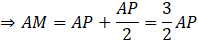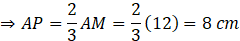Question 27.

In a trapezium ABCD, seg AB || seg DC seg BDseg AD, seg ACseg BC, If AD = 15, BC = 15 and AB = 25. Find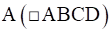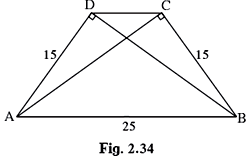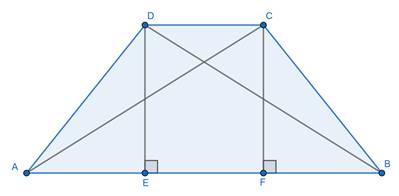Construct DE ⊥ AB and CF ⊥ AB

(Hypotenuse)2 = (base)2 + (Perpendicular)2

⇒ 252 = 152 + BD2

⇒ BD2 = 625 - 225 = 400

⇒ BD = 20 cm

Similarly,

AC = 20 cm

Now, In ΔAED and ΔABD

∠DAE = ∠DAE [Common]

ΔAED ~ ΔABD [By Angle-Angle Criteria]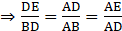[Property of similar triangles]

As AD = 15 cm, BD = 20 cm and AB = 25 cm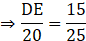⇒ DE = 12 cm

Also,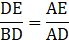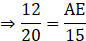⇒ AE = 9 cm

Similarly, BF = 9 cm

Now,

DC = EF [By construction]

DC = AB - DE - AE

DC = 25 - 9 - 9 = 7 cm

Also, we know

Area of trapezium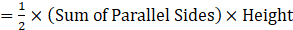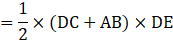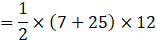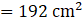Question 28.

In the figure 2.35, ∆ P20QR is an equilatral triangle. Point S is on seg QR such that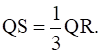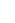Prove that : 9 PS2 = 7 PQ2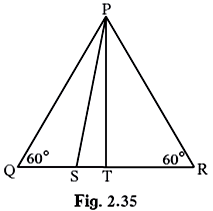As, PQR is an equilateral triangle,

Point S is on base QR, such that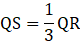PT is perpendicular on side QR from P.

As, PQR is an equilateral triangle we have

PQ = QR = PR 

Also, we know that Perpendicular from a vertex to corresponding side in an equilateral triangle bisects the side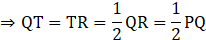Now, In ΔPTQ, By Pythagoras theorem

(Hypotenuse)2 = (base)2 + (Perpendicular)2

⇒ PQ2 = PT2 + QT2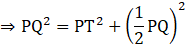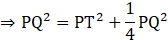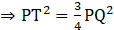As,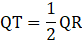We have,

QT - QS = ST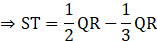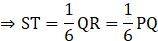Now, In right angled triangle PST, By Pythagoras theorem

(PS)2 = (ST)2 + (PT)2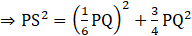[From 2]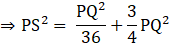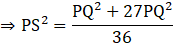⇒ 36 PS2 = 28 PQ2

⇒ 9 PS2 = 7 PQ2

Hence Proved.

Question 29.

Seg PM is a median of ∆ PQR. If PQ = 40, PR = 42 and PM = 29, find QR.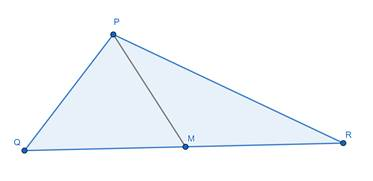We know, By Apollonius theorem

In ΔPQR, if M is the midpoint of side QR, then PQ2 + PR2 = 2PM2 + 2QM 2

Given that, PM is median i.e. M is the mid-point of QR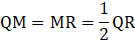And PQ = 40, PR = 42, PM = 29

Putting values,

⇒ (40)2 + (42)2 = 2(29)2 + 2(QM)2

⇒ 1600 + 1764 = 1682 + 2QM2

⇒ QM2 = 1682

⇒ QM = 29

⇒ QR = 2(29) = 58

Question 30.

Seg AM is a median of ∆ ABC. If AB = 22, AC = 34, BC = 24, find AM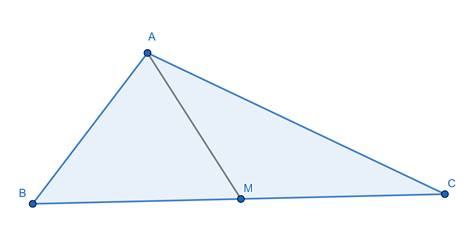We know, By Apollonius theorem

In ΔABC, if M is the midpoint of side BC, then AB2 + AC2 = 2AM2 + 2BM 2

Given that,

AB = 22, AC = 34, BC = 24

AP is median i.e. P is the mid-point of BC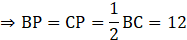Putting values in equation

⇒ 222 + 342 = 2AM2 + 2(12)2

⇒ 484 + 1156 = 2AM2 + 288

⇒ 1352 = 2AM2

⇒ AM2 = 676

⇒ AM = 26

## PDF FILE TO YOUR EMAIL IMMEDIATELY PURCHASE NOTES & PAPER SOLUTION. @ Rs. 50/- each (GST extra)

SUBJECTS

HINDI ENTIRE PAPER SOLUTION

MARATHI PAPER SOLUTION
SSC MATHS I PAPER SOLUTION
SSC MATHS II PAPER SOLUTION
SSC SCIENCE I PAPER SOLUTION
SSC SCIENCE II PAPER SOLUTION
SSC ENGLISH PAPER SOLUTION
SSC & HSC ENGLISH WRITING SKILL
HSC ACCOUNTS NOTES
HSC OCM NOTES
HSC ECONOMICS NOTES
HSC SECRETARIAL PRACTICE NOTES

2019 Board Paper Solution

HSC ENGLISH SET A 2019 21st February, 2019

HSC ENGLISH SET B 2019 21st February, 2019

HSC ENGLISH SET C 2019 21st February, 2019

HSC ENGLISH SET D 2019 21st February, 2019

SECRETARIAL PRACTICE (S.P) 2019 25th February, 2019

HSC XII PHYSICS 2019 25th February, 2019

CHEMISTRY XII HSC SOLUTION 27th, February, 2019

OCM PAPER SOLUTION 2019 27th, February, 2019

HSC MATHS PAPER SOLUTION COMMERCE, 2nd March, 2019

HSC MATHS PAPER SOLUTION SCIENCE 2nd, March, 2019

SSC ENGLISH STD 10 5TH MARCH, 2019.

HSC XII ACCOUNTS 2019 6th March, 2019

HSC XII BIOLOGY 2019 6TH March, 2019

HSC XII ECONOMICS 9Th March 2019

SSC Maths I March 2019 Solution 10th Standard11th, March, 2019

SSC MATHS II MARCH 2019 SOLUTION 10TH STD.13th March, 2019

SSC SCIENCE I MARCH 2019 SOLUTION 10TH STD. 15th March, 2019.

SSC SCIENCE II MARCH 2019 SOLUTION 10TH STD. 18th March, 2019.

SSC SOCIAL SCIENCE I MARCH 2019 SOLUTION20th March, 2019

SSC SOCIAL SCIENCE II MARCH 2019 SOLUTION, 22nd March, 2019

XII CBSE - BOARD - MARCH - 2019 ENGLISH - QP + SOLUTIONS, 2nd March, 2019

HSC Maharashtra Board Papers 2020

(Std 12th English Medium)

HSC ECONOMICS MARCH 2020

HSC OCM MARCH 2020

HSC ACCOUNTS MARCH 2020

HSC S.P. MARCH 2020

HSC ENGLISH MARCH 2020

HSC HINDI MARCH 2020

HSC MARATHI MARCH 2020

HSC MATHS MARCH 2020

SSC Maharashtra Board Papers 2020

(Std 10th English Medium)

English MARCH 2020

HindI MARCH 2020

Hindi (Composite) MARCH 2020

Marathi MARCH 2020

Mathematics (Paper 1) MARCH 2020

Mathematics (Paper 2) MARCH 2020

Sanskrit MARCH 2020

Important-formula

THANKS